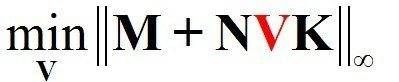# How to Minimize the infinity norm of a matrix function

• rinna

#### rinna

Hi , I have been thinking of this question for a long time. Can someone give me an advice?

There are three known matrices M, N, and K.

M is a (4*4) matrix:

M=
[ 1 0 2 3;
2 1 3 5;
4 1 1 2;
0 3 4 3 ]

N is a (4*3) matrix:

N=
[ 3 0 4;
1 5 2;
7 1 3;
2 2 1 ]

K is a (2*4) matrix:

K=
[ 1 0 2 3;
2 1 3 5 ]

There is an unknown matrix V, whose size is (3*2).

My question is:

How to minimize the infinity norm of M+NVK and find the V which can minimize the infinity norm of the matrix function? the question formulation is shown in the following:Welcome to PF, Rinna!I'm not aware of a method to solve this algebraically.
So I would solve it numerically.
You can do this with most programs that support minimization.

I just did that and found the minimum 5.389.

You could get it down to 5.0 just by making each entry of V equal to zero.

You could get it down to 5.0 just by making each entry of V equal to zero.

With V=0 we get the infinity norm of matrix M, which is |2| + |1| + |3| + |5| = 11.
How did you get 5.0?

I interpret the infinity norm to be max{|2|,|1|,|3|,|5|} = 5.

I started looking on the web too. Planet Math (under "Matrix p-norm") defines the infinity norm to be the maximum of a set of sums. Each sum is the sum of the absolute values in a row of the matrix. I trust that reference the most.

Using any definitions we have encountered, the problem is at least as simple as solving a collection of linear programming problems. The objective function (the infinity norm) is going to involve one or more absolute values of linear functions of the entries of V. One may split the problem into cases by assuming a sign for each expression inside an absolute value sign. This produces linear constraints. For example, if we assume an expression is postiive then the linear function inside the absolute value sign is greater than zero. If there is a max{...} involved, we define cases by considering the possibility that each individual expression in the {...} is the max. This gives a set of inequalities that say that expression is greather than the others. These are also linear constraints.

Last edited:
I started looking on the web too. Planet Math (under "Matrix p-norm") defines the infinity norm to be the maximum of a set of sums. Each sum is the sum of the absolute values in a row of the matrix. I trust that reference the most.

I think you just agreed with me here.

Using any definitions we have encountered, the problem is at least as simple as solving a collection of linear programming problems. The objective function (the infinity norm) is going to involve one or more absolute values of linear functions of the entries of V. One may split the problem into cases by assuming a sign for each expression inside an absolute value sign. This produces linear constraints. For example, if we assume an expression is postiive then the linear function inside the absolute value sign is greater than zero. If there is a max{...} involved, we define cases by considering the possibility that each individual expression in the {...} is the max. This gives a set of inequalities that say that expression is greather than the others. These are also linear contraints.

Yes, this would lead to 4 inequalities, each containing 4 absolute values, with 6 unknowns.
Since each absolute value can imply 2 signs, this results in 16 possibilities (linear expressions) for each inequality.

The resulting system looks to be prohibitively complex to solve for a mere human.

I think you just agreed with me here.

I agree that I agreed with you!

Last edited by a moderator: